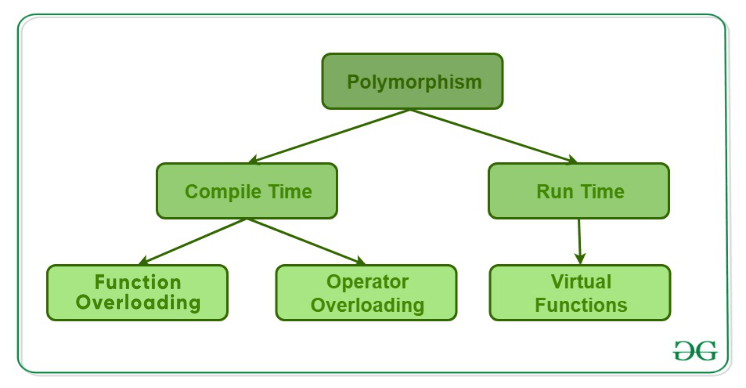# Polymorphism in C++

• Difficulty Level : Easy
• Last Updated : 22 Jun, 2022

The word “polymorphism” means having many forms. In simple words, we can define polymorphism as the ability of a message to be displayed in more than one form. A real-life example of polymorphism is a person who at the same time can have different characteristics. Like a man at the same time is a father, a husband and an employee. So the same person exhibits different behavior in different situations. This is called polymorphism. Polymorphism is considered as one of the important features of Object-Oriented Programming. In C++, polymorphism is mainly divided into two types:

• Compile-time Polymorphism
• Runtime PolymorphismTypes of Polymorphism

• Function Overloading: When there are multiple functions with the same name but different parameters, then the functions are said to be overloaded. Functions can be overloaded by changing the number of arguments or/and changing the type of arguments. Rules of Function Overloading

## CPP

 `// C++ program for function overloading` `#include `   `using` `namespace` `std;` `class` `Geeks` `{` `    ``public``:` `    `  `    ``// function with 1 int parameter` `    ``void` `func(``int` `x)` `    ``{` `        ``cout << "value of x is " << x << endl;` `    ``}` `    `  `    ``// function with same name but 1 double parameter` `    ``void` `func(``double` `x)` `    ``{` `        ``cout << "value of x is " << x << endl;` `    ``}` `    `  `    ``// function with same name and 2 int parameters` `    ``void` `func(``int` `x, ``int` `y)` `    ``{` `        ``cout << "value of x and y is " << x << ", " << y << endl;` `    ``}` `};`   `int` `main() {` `    `  `    ``Geeks obj1;` `    `  `    ``// Which function is called will depend on the parameters passed` `    ``// The first 'func' is called ` `    ``obj1.func(7);` `    `  `    ``// The second 'func' is called` `    ``obj1.func(9.132);` `    `  `    ``// The third 'func' is called` `    ``obj1.func(85,64);` `    ``return` `0;` `} `

• Output:
```value of x is 7
value of x is 9.132
value of x and y is 85, 64```
• In the above example, a single function named func acts differently in three different situations, which is a property of polymorphism.
• Operator Overloading: C++ also provides the option to overload operators. For example, we can make use of the addition operator (+) for string class to concatenate two strings. We know that the task of this operator is to add two operands. So a single operator ‘+’, when placed between integer operands, adds them and when placed between string operands, concatenates them. Example

## CPP

 `// CPP program to illustrate` `// Operator Overloading` `#include` `using` `namespace` `std;` ` `  `class` `Complex {` `private``:` `    ``int` `real, imag;` `public``:` `    ``Complex(``int` `r = 0, ``int` `i =0)  {real = r;   imag = i;}` `     `  `    ``// This is automatically called when '+' is used with` `    ``// between two Complex objects` `    ``Complex operator + (Complex ``const` `&obj) {` `         ``Complex res;` `         ``res.real = real + obj.real;` `         ``res.imag = imag + obj.imag;` `         ``return` `res;` `    ``}` `    ``void` `print() { cout << real << " + i" << imag << endl; }` `};` ` `  `int` `main()` `{` `    ``Complex c1(10, 5), c2(2, 4);` `    ``Complex c3 = c1 + c2; ``// An example call to "operator+"` `    ``c3.print();` `}`

• Output:
`12 + i9`
• In the above example, the operator ‘+’ is overloaded. Usually, this operator is used to add two numbers (integers or floating point numbers), but here the operator is made to perform the addition of two imaginary or complex numbers. To learn about operator overloading in detail, visit this link.
1. Runtime polymorphism: This type of polymorphism is achieved by Function Overriding.
• Function overriding occurs when a derived class has a definition for one of the member functions of the base class. That base function is said to be overridden

## CPP

 `// C++ program for function overriding`   `#include ` `using` `namespace` `std;`   `class` `base` `{` `public``:` `    ``virtual` `void` `print ()` `    ``{ cout<< "print base ``class``" <print(); ` `     `  `    ``// Non-virtual function, binded at compile time` `    ``bptr->show(); `   `    ``return` `0;` `} `

• Output:
```print derived class
show base class```